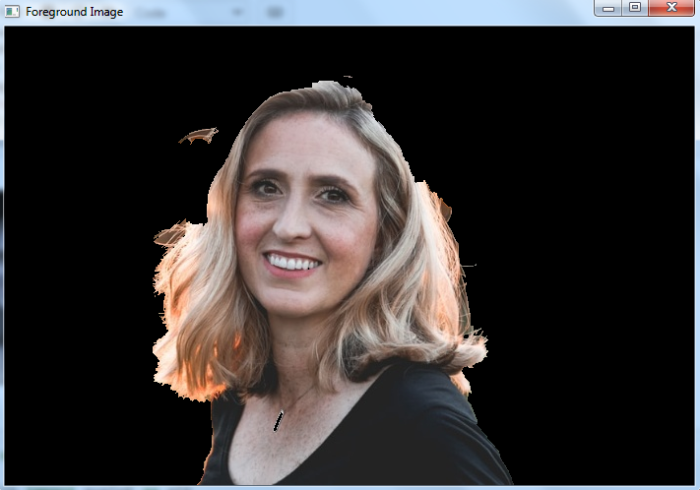# How to extract the foreground of an image using OpenCV Python?

We apply the cv2.grabCut() method to extract the foreground in an image. For detailed approach please follow the steps given below −

• Import the required libraries OpenCV and NumPy. Make sure you have already installed them

• Read the input image using cv2.imread() method. Specify the full image path.

• Define the variables: mask, bgdModel and fgdModel.

• Define the coordinates of a rectangle "rect" which includes the foreground object in the format (x,y,w,h). The correct coordinates are very important to extract the meaningful foreground.

• Apply grabCut() algorithm to extract the foreground of the input image. Pass mask, rect, bgdModel, fgdModel, iterCount and mode to the algorithm as parameters. We apply mode as cv2.GC_INIT_WITH_RECT as we use the rectangle.

cv2.grabCut(img,mask,rect,bgdModel,fgdModel,iterCount,cv2.GC_IN IT_WITH_RECT)

• Obtain the new mask mask2. Multiply the new mask with the image to find the segmented image (foreground).

• Display the extracted foreground.

Let's have a look at a program example for a better understanding.

## Input Image

We will use the following image as an input file in the example below.## Example

In this example, we extract the foreground in the input image.

# import required libraries
import numpy as np
import cv2

# from matplotlib import pyplot as plt

bgdModel = np.zeros((1,65),np.float64)
fgdModel = np.zeros((1,65),np.float64)

# define rectangle
rect = (150,50,500,470)

# apply grabCut method to extract the foreground

# display the extracted foreground image

# plt.imshow(img),plt.colorbar(),plt.show()
cv2.imshow('Foreground Image',img)
cv2.waitKey(0)
cv2.destroyAllWindows()


## Output

When we execute the above program, it will produce the following output window.We use iterCount as 20 in the above program code. You can adjust the number of iterations the algorithm should run for better results. Also for better results the rectangle coordinates (rect mentioned in the example program) are very important. We use rect = (150,50,500,470) as our foreground lies within these coordinates. Change these coordinates according to the input image.

Updated on: 02-Dec-2022

2K+ Views